# Appell transformation

A transformation of the equations of plane motion of a material point under the action of a force that varies with the coordinatesof the point only. The Appell transformation is a homographic transformation of the coordinatesinto the coordinates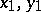:which involves the substitution of the time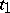forin accordance with the formula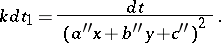As a result of the Appell transformation, the motion of a point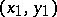, acted upon by a force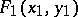that depends only on the coordinates, corresponds to the motion of the point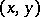acted upon by the force. A transformation of the equations of motion of a pointwith the aid of formulas of general form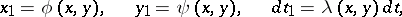(*)

yields the equations of motion of the pointunder the effect of a force that depends only on the coordinatesonly if (*) is a homographic transformation. The Appell transformation is used to solve Bertrand's problem on the determination of the forces under which the trajectories of motion of material points become conic sections. The Appell transformation is named after P.E. Appell.